+

# Ratio Analysis

Author: Sophia Tutorial
##### Description:

Calculate a profitability ratio or a liquidity ratio.

(more)### Developing Effective Teams

*No strings attached. This college course is 100% free and is worth 1 semester credit.

37 Sophia partners guarantee credit transfer.

299 Institutions have accepted or given pre-approval for credit transfer.

* The American Council on Education's College Credit Recommendation Service (ACE Credit®) has evaluated and recommended college credit for 32 of Sophia’s online courses. Many different colleges and universities consider ACE CREDIT recommendations in determining the applicability to their course and degree programs.

Tutorial
what's covered
This tutorial will cover the topic of ratio analysis.

Our discussion breaks down as follows:

1. Profitability Ratios
1. Rate of Return on Sales
2. Return on Total Assets
3. Asset Turnover Ratio
2. Liquidity Ratios
1. Current Ratio
2. Inventory Turnover

## 1. Profitability Ratios

Before we begin our discussion on ratio analysis, it's important to have an understanding of a financial ratio, which evaluates the relationship between specific items on a financial statement.

The first step of ratio analysis is calculating our profitability ratios, which measure the operating performance of a company, helping a business to understand and evaluate its performance.

Today we will be covering the following profitability ratios:

Profitability Ratio Measurement
Rate of return on sales Measures managerial efficiency, as well as profitability
Return on total assets Measures the effective use of assets and managerial efficiency
Asset turnover Measures the use of assets to make sales

term to know

Financial Ratio
Evaluates the relationship between specific items on a financial statement.

1a. Rate of Return on Sales
The formula for rate of return on sales is net income divided by net sales. This ratio will tell us how profitable we are as a business.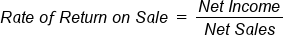Suppose net income is \$257,500 and net sales is \$925,000, we would get a rate of return on sales of 27.8%.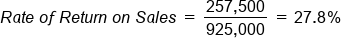1b. Return on Total Assets
Next, the formula for return on total assets is income before interest expense and taxes, divided by total assets. This ratio is going to tell us how effectively we use our assets to generate income.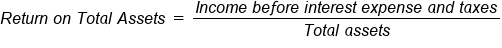Suppose the income before interest expense and taxes is \$262,500 and total assets is \$980,000, then we get a return on total assets of 26.8%.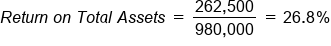1c. Asset Turnover Ratio
Lastly, the formula for asset turnover ratio is net sales divided by total assets. This ratio helps us measure the use of assets to make sales.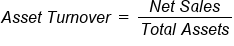Suppose net sales is \$925,000 and total assets is \$980,000, we can see that our assets turn over 0.9 times during the year.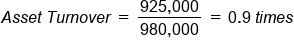## 2. Liquidity Ratios

Now that we've learned how to calculate profitability ratios, let's turn our attention to liquidity ratios. Liquidity ratios measure the ability of a company to pay debts when they are due. In other words, they help us understand our ability to pay our debt obligations.

Today we will discuss the following liquidity ratios:

Liquidity Ratio Measurement
Current Ratio Measures how much in current assets a company has to pay its current liabilities
Inventory Turnover Measures the number of times a company's inventory is sold and replaced

2a. Current Ratio
The formula for current ratio is current assets divided by current liabilities.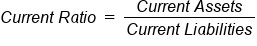Suppose that current assets is \$580,000 and current liabilities is \$155,000, we get a current ratio of 3.74, which means we have 3.74 times more current assets than we do current liabilities.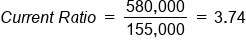2b. Inventory Turnover
Now let's turn our attention to inventory turnover. Inventory turnover is calculated as cost of goods sold divided by average inventory.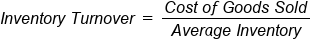Suppose cost of goods sold is \$450,000 and average inventory is \$225,000, we see that inventory turnover is 2.0 times. This is the number of times the inventory is sold and replaced.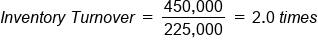It's important to note that in order for these liquidity ratios to have context, we would need to know information such as what our ratios were last year, other companies' ratios, and the industry standards.

summary
Today we learned how to perform ratio analysis. We learned how to calculate profitability ratios, looking at rate of return on sales, return on total assets, as well as asset turnover. W also learned how to calculate liquidity ratios by looking at current ratio and inventory turnover.

Source: Adapted from Sophia instructor Evan McLaughlin.

Terms to Know
Financial Ratio

Evaluates the relationship between specific items on a financial statement.

Rating